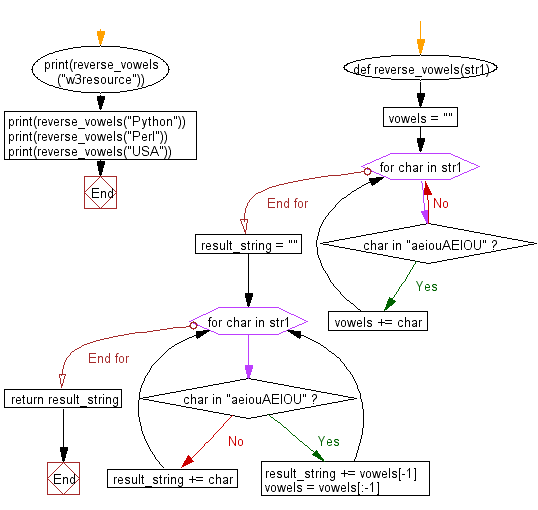﻿ Python: Reverse only the vowels of a given string - w3resource# Python: Reverse only the vowels of a given string

## Python Basic - 1: Exercise-71 with Solution

Write a Python program to reverse only the vowels of a given string.

Sample Solution:

Python Code:

``````def reverse_vowels(str1):
vowels = ""
for char in str1:
if char in "aeiouAEIOU":
vowels += char
result_string = ""
for char in str1:
if char in "aeiouAEIOU":
result_string += vowels[-1]
vowels = vowels[:-1]
else:
result_string += char
return result_string
print(reverse_vowels("w3resource"))
print(reverse_vowels("Python"))
print(reverse_vowels("Perl"))
print(reverse_vowels("USA"))
``````

Sample Output:

```w3resuorce
Python
Perl
ASU
```

Pictorial Presentation:Flowchart:Python Code Editor:

Have another way to solve this solution? Contribute your code (and comments) through Disqus.

What is the difficulty level of this exercise?

Test your Programming skills with w3resource's quiz.

﻿

## Python: Tips of the Day

What is the difference between Python's list methods append and extend?

append: Appends object at the end.

```x = [1, 2, 3]
x.append([4, 5])
print (x)
```

Output:

```[1, 2, 3, [4, 5]]
```

extend: Extends list by appending elements from the iterable.

```x = [1, 2, 3]
x.extend([4, 5])
print (x)
```

Output:

```[1, 2, 3, 4, 5]
```

Ref: https://bit.ly/2AZ6ZFq